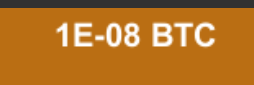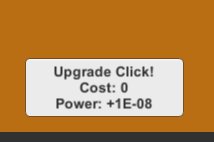# Problem with upgrade and long numbers

1.NumbersCode:

``````using System.Collections;
using UnityEngine;

public class ClickUpgrade : MonoBehaviour {

public Click click;
public UnityEngine.UI.Text itemInfo;
public float cost;
public int count = 0;
public float clickPower;
public string itemName;
private float _newCost;

void Update()
{
itemInfo.text = itemName + "
``````

Cost: " + cost + "
Power: +" + clickPower;
}

``````    public void PurchasedUpgrade()
{
if (click.BTC >= cost)
{
click.BTC -= cost;
count += 1;
click.perclick += clickPower;
cost = Mathf.Round(cost * 2);
_newCost = Mathf.Pow (cost, _newCost = cost);
}
}
}
``````

``````cost = Mathf.Round(cost * 2);
So if the initial value of `cost` is less than 0.5, then `Mathf.Round` makes it “0”.